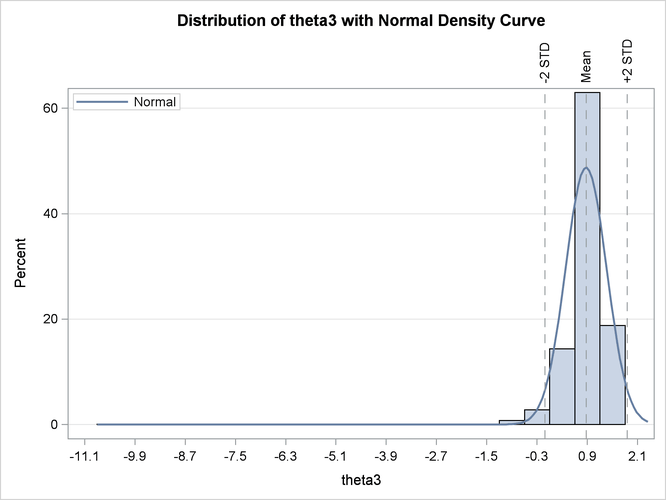The NLIN Procedure

Example 69.7 Parameter Profiling and Bootstrapping

This example, which uses a model from Clarke (1987), shows why it is difficult to intuitively reason about the characteristics of the parameters that occur in a nonlinear model. As a consequence, the example demonstrates the indispensability of parameter profiling and confidence curves to identify the nonlinear characteristics of model parameters. The parameter profiling is also augmented with a plot that shows the influence of each observation on the parameter estimates. Furthermore, the BOOTSTRAP statement in PROC NLIN is invoked in order to study the sampling distribution of parameter estimates and make more accurate statistical inferences.

The model takes the form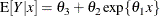The data set in this example is from Clarke (1987). The following DATA step creates this data set:

data clarke1987a;
input  x y;
datalines;
1    3.183
2    3.059
3    2.871
4    2.622
5    2.541
6    2.184
7    2.110
8    2.075
9    2.018
10   1.903
11   1.770
12   1.762
13   1.550
;


The model is fit by using the following statements in the NLIN procedure:

ods graphics on;
proc nlin data=clarke1987a plots(stats=none)=diagnostics;
parms theta1=-0.15
theta2=2.0
theta3=0.80;
profile theta1 theta3 / range = -6 to 2 by 0.2 all;
bootstrap / nsamples = 2000 seed=123 bootplots bootci bootcov;
model y = theta3 + theta2*exp(theta1*x);
run;
ods graphics off;


Output 69.7.1: Parameter Estimates and Bootstrap Estimate of the Covariance Matrix

The NLIN Procedure

Parameter Estimate Approx
Std Error
Approximate 95% Confidence
Limits
Bootstrap
Std Dev
Bootstrap Bias-Corrected
95% Confidence Limits
theta1 -0.1031 0.0255 -0.1599 -0.0462 0.0253 -0.1505 -0.0531
theta2 2.5190 0.2658 1.9268 3.1112 0.4450 2.1716 3.5325
theta3 0.9631 0.3216 0.2466 1.6797 0.4907 -0.2565 1.3697

Bootstrap Covariance Matrix Estimate
theta1 theta2 theta3
theta1 0.00063801 0.00834138 -.01006296
theta2 0.00834138 0.19802119 -.21570467
theta3 -.01006296 -.21570467 0.24082392

By default, the BOOTCI option in the BOOTSTRAP statement adds a column that contains the bias-corrected bootstrap confidence limits to the "Parameter Estimates" table (Output 69.7.1). The BOOTCOV option produces the "Bootstrap Covariance Matrix" table (Output 69.7.1). The discrepancy between the Wald-based confidence limits and the bias-corrected bootstrap confidence limits is much greater for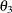than for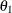. The profile t plot, confidence curve, and histograms of the bootstrap estimates show why this is the case.

The profile t plot for parameterin Output 69.7.3 shows a definite deviation from the linear reference line that has a slope of 1 and passes through the origin. Hence, Wald-based inference foris not appropriate. In contrast, the profile t plot for parameterin Output 69.7.2 shows that Wald-based inference formight be sufficient.

Output 69.7.4 and Output 69.7.5 show the confidence curves forand, respectively. For, you can see a significant difference between the Wald-based confidence interval and the corresponding likelihood-based interval. In such cases, the likelihood-based intervals are preferred because their coverage rate is much closer to the nominal values than the coverage rate of the Wald-based intervals (Donaldson and Schnabel, 1987; Cook and Weisberg, 1990).

Output 69.7.6 depicts the influence of each observation on the value of. Observations 6 and 13 have the most influence on the value of this parameter. The plot is generated using the leave-one-out method and should be contrasted with the local influence plot in Output 69.7.7, which is based on assessing the influence of an additive perturbation of the response variable.

Output 69.7.8 and Output 69.7.9 are histograms that show the distribution of the bootstrap parameter estimates forand, respectively. These histograms complement the information that is obtained aboutandfrom the profile t plots. Specifically, they show that the bootstrap parameter estimate ofhas a distribution close to normal, whereas that ofhas a distribution that deviates significantly from normal. Again, this leads to the conclusion that inferences based on linear approximations, such as Wald-based confidence intervals, work better forthan for.

Finally, this example shows that the adequacy of a linear approximation with regard to a certain parameter cannot be inferred directly from the model. If it could, then, which enters the model linearly, would have a completely linear behavior, whereaswould have a highly nonlinear behavior. However, the diagnostics that are based on the profile t plot and confidence curves, and the histograms of the bootstrap parameter estimates, show that the opposite holds. For a detailed discussion about this issue, see Cook and Weisberg (1990).

Output 69.7.2: Profile t Plot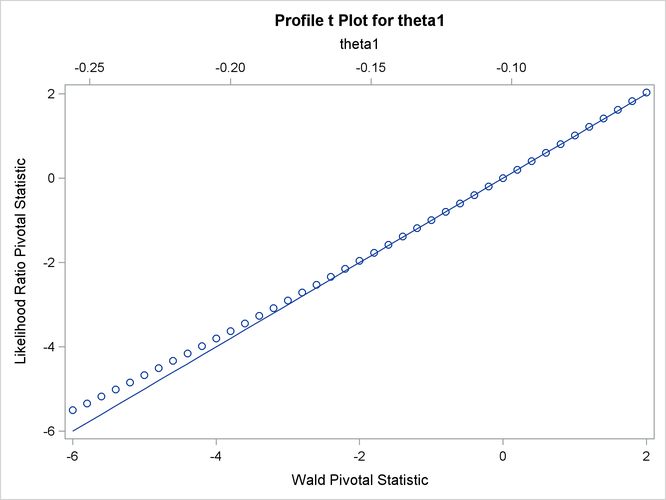Output 69.7.3: Profile t Plot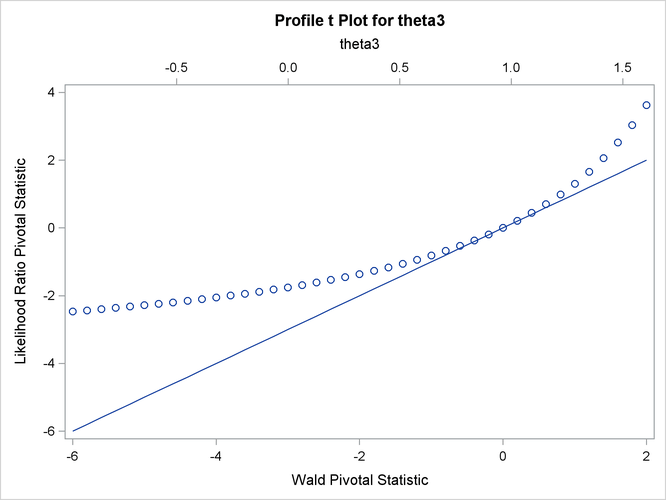Output 69.7.4: Confidence Curve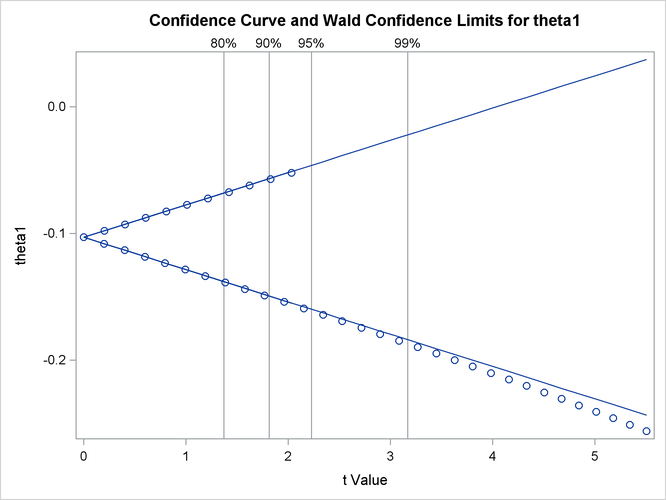Output 69.7.5: Confidence Curve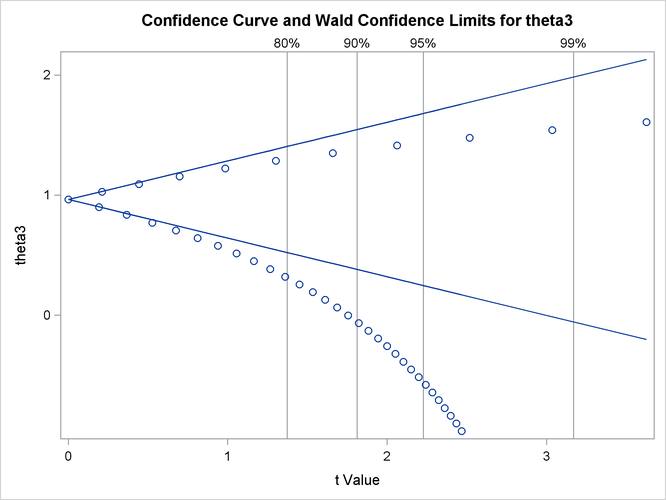Output 69.7.6: Observation Influence on Parameter Estimate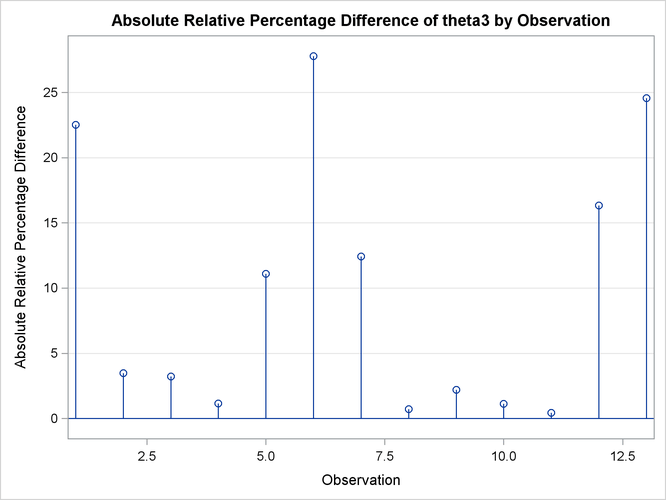Output 69.7.7: Local Influence Plot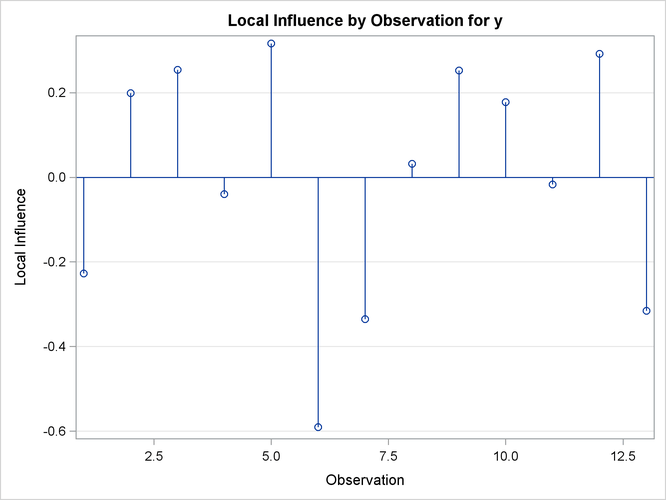Output 69.7.8: Histogram of Bootstrap Parameter Estimates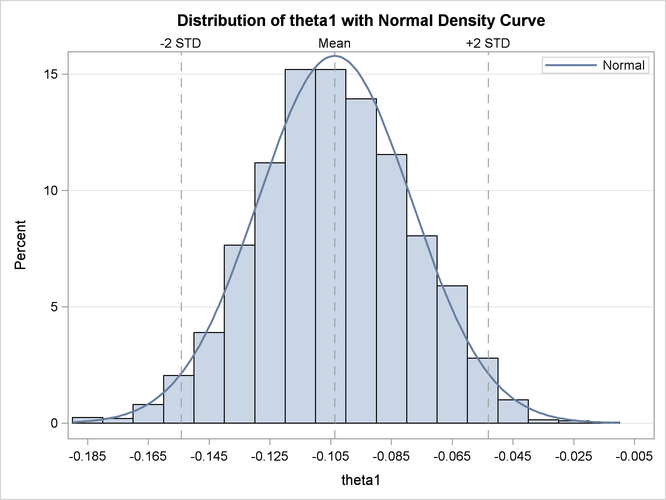Output 69.7.9: Histogram of Bootstrap Parameter Estimates